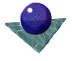Rotating, translating and
estimating pose of a pyramidFirst, define a pyramid illustrated in Figure 3, below, by the five vertex points

x = ((1,1,0),(1,-1,0),(-1,-1,0),(-1,1,0),( 0,0,1))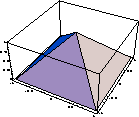Figure 3:   A pyramid used as a test object

Then consider a rotation of 45 degrees about the axis,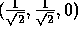. Using Equation 12 the rotation matrix is (to two decimal places )Rotation of the pyramid using the rotation matrix defined above gives the vertex coordinates,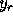= ((1,1,0),(0.71,-0.71,-1),(-1,-1,0),(-0.71,0.71,1),(0.50,-0.50,0.71) The resultant pyramid is illustrated in Figure 4, below.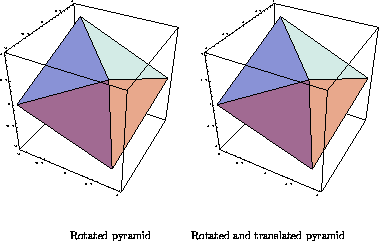Figure 4:   Transformations of the square pyramid

Then we can translate the rotated pyramid by a translation vector (1,2,3) to give a new pts list, also illustrated in Figure 4.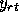= ((2,3,3),(1.71,1.29,2),(0,1,3),(0.29,2.71,4),(1.50,1.50,3.71)) Then we can use the Least Squares technique to calculate the rotation matrix, given the control points which define the vertices in Figures 3 and 4. This recovers the original rotation matrix Equation 26, above) and translation defined above with no residual error.

Then, we can add random errors to the list of scene points,, for example,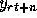= (2.10,3.05,3.20),(1.77,1.28,2.05),(0.02,1.00,2.87),(0.29,2.91,3.99),(1.51,1.48,3.78)) When the pose estimate is recomputed, we now obtain a different rotation matrix, a different translation vector, and a finite residual error.and translation vector, (1.056,2.05,3.03), with a mean square residual error between the rotated model and scene data of (0.004,0.032,0.009).Comments to: Sarah Price at ICBL.